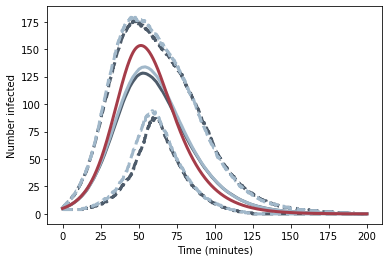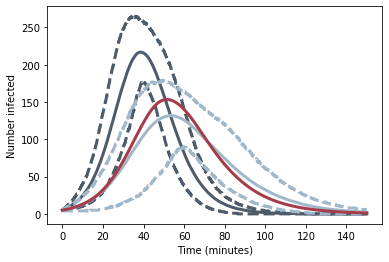Search

# Playground Pox

This is not a COVID model, or even an epidemic model. It's a toy model of a playground, designed to illustrate aspects of serious models of a global epidemic.It is the model I used to produce the figures used in my "Pains of epidemiology" article, to which this blog acts as a slightly nerdy companion piece.

For those kind souls who have asked, I will write a version of this with equations. In the meantime, send me a mail if you would like a copy of the code (Python).

## Introduction

This is a snapshot from Playground Pox - a game our eleven-year old son created in Snap the evening before the Danish state sent him back to school. The picture links to a film.

The 20 children in Theo's class are playing in the school playground. One of them is infected with the Pox. Every time an infected child interacts with an uninfected child, there is a certain probability that the infection is transmitted. The children are infected for a random length of time, after which they recover and are immune.

With such a small population, the dynamics of this (brief) contagion are very unstable. Sometimes everyone is infected and becomes immune. Sometimes, child zero recovers before he has managed to infect anyone and sometimes a measure of herd immunity obtains leaving some proportion of the class uninfected and the rest immune.This figure shows twenty different runs, all with the same probability of infection. The vertical axis shows the number of kids as yet uninfected as a function of time. The horizontal axis is time. The thick, dark curve is the average of the twenty runs, which remarkably fails to capture any of the realized instances. The span of final outcomes - from no infections to all but one of the class getting infected - is equally remarkable.

Modelling this toy example encounters many of the fundamental challenges faced by serious epidemiological models, but in a simple, manageable setting. In this article, I will outline several ways of modelling Playground Pox that correspond to the kinds of models presented in the literature on epidemiological modelling of the COVID pandemic.

Obviously Pox models are too, well, poxy to be applied to COVID, but my hope is that they nevertheless provide some insight into the models that are having such a profound influence on our every day lives today.

## The "full" model

In order that some of the approximate models we later build have any chance of working, we'll need some more kids. Let's say we have a big playground with 1000 children.

Every child on the playground has access to every other child on the playground, but we'll assume the school is grouped into classes with 20 kids and year groups made up of five classes. Within classes the kids have potentially infectious contact, say, 25 times an hour on average; between classes in the same year, 5 times an hour; and between years, once an hour. These contact events will be where the infection is transmitted.

The actual number of contacts each child has with each other child in the course of a given hour is a random variable, which we model with a Poisson distribution parameterized with the average number of contacts between the two children. We focus on the interactions between those infected and those uninfected.

If the number of infectious contacts an uninfected child has is n and each time there is an infectious contact, there is a probability p of infection, then the probability that the child is not infected at the end of the hour is (1-p)^n, so the probability he is infected at the end of the hour is 1-(1-p)^n. If p is small, this is very close to np, which makes intuitive sense (though it really ought not)

Once a child is infected, he stays infected for a random length of time, which is modelled by a Gamma distribution, with mean 15 minutes and standard deviation 5 minutes. During this time, he can infect other uninfected children. After this time, he recovers, stops infecting other kids and becomes immune.The output from such a model is shown here, starting with five kids in a single class infected. The solid lines are the means of the number of uninfected (dark blue), infected (red) and recovered (light blue) kids as a function of time. The dashed lines are the 10th and 90th percentiles and the thin lines are realizations. Even with this many kids, there are wide range of outcomes, though this is in part owing to the relatively small number of initial infectious cases. Once the contagion gets going, it's relatively predictable.

## A fast stochastic model for a homogeneous playground

The full model has to keep track of all the kids - in particular whether or not they are infectious in any given minute (second, actually, in practice) - so it's rather slow.

I can speed things up a lot if I give up on specifying the contact rates between all the individual kids and just replace them by their average.

The total number of infectious contacts for each child in a given minute is the sum of all the individual infectious contacts with the other children, which are Poisson distributed with a now constant mean rate. The total, too, is Poisson distributed with a mean that is the average contact rate times the number of infected kids.

If the number of infected kids is large, then the variance of the distribution of the total number of contacts around this mean is relatively small. As such, the variance of probability of each uninfected child getting infected (as given above) is also small.

If all the uninfected kids have roughly the same probability of getting infected, then the number of kids infected in a given hour is binomially distributed with probability the probability each child gets infected (roughly the number of infected kids multiplied by the average number of contacts for each kid multiplied by the probability of transmission in an infected / uninfected contact) and number of samples the number of as yet uninfected kids.

I can make a similar simplification if I assume that the probability that an infected child recovers is constant for all the children. This clearly isn't the case as the probability any given child recovers in a given time period will depend on how long they've been ill and the distribution of those probabilities will change over time. If the distribution of times for which the kids are infected is exponential then the probability of exiting in any given time period is that same for all the infected kids owing to the memoryless property of the exponential distribution.

In this case, the number of kids recovering in a given minute is again binomially distributed with probability the probability the child recovers in that minute given that it hasn't yet recovered and the number of samples the number of infected kids.

## SIR

The simplest Susceptible - Infectious - Recovered model is essentially identical to the fast stochastic model. The expected number of people transitioning from susceptible to infectious in a given time step in the fast stochastic model is the expected value of the binomial distribution describing the number of people transferring in a given time step. This is the number of uninfected kids multiplied by the probability each child gets infected, which in turn is (roughly) the number of infected kids multiplied by the average number of contacts per child per time step multiplied by the probability of transmission). A similar argument applies to the expected number of people transitioning from infectious to recovered.

Both these expressions are identical to the expressions for the rate of flux between compartments in the SIR model. As such SIR can be thought of as a model of the expected (ensemble averaged) numbers in the limit of small time steps.

## ComparisonsWe can compare SIR and the fast model with the full model if we set the infectious time distribution in the full model to exponential and set all the contact rates between children to a constant value. The results are shown here with the full model in the same colour as before, the fast model in blue and SIR in red. The fast model works pretty well, both in terms of assessing the mean and the uncertainty ranges (dotted lines), showing that for this size of population at least the approximations regarding the number of infectious contacts are reasonable. SIR, as expected, tracks the mean result of fast model. The deviation is due to the fact that the SIR model is integrated using a fourth order method, rather than the one-step Euler method the analogy implies.What about the exponential distribution and the inhomogenous population. If we first leave the fast model as it is and return the full model to a Gamma distribution (but leave it with a homogeneous population) we get this figure. SIR is still slavishly tracking the fast model, but clearly the distribution of times spent infectious makes a substantial difference to the overall outcome.We now switch back to an exponential infectious time distribution in order compare the effect of averaging the contact rates relative to specifying the full contact structure in this simple case. Again, SIR and the fast model continue their close collaboration, but the importance of contact structure is pronounced.

See All